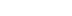/

# The Trials and Tribulations of Tribology

The simple force of friction in the physical world is so familiar to everyone in a potential game audience, that any departure from realism can be glaring and yet realistically simulating these simple physical properties is quite challenging. In this article Jeff Lander discusses the simulation of friction in real-time 3D applications, otherwise known as the field of tribology.

I have decided that friction is a drag. It's almost as easy to understand as gravity. We deal with it every day. Friction keeps me from sliding completely under my desk when I slouch in my chair. It keeps my car from spinning out of control as I turn corners with reckless abandon.

This experience with friction begins when as babies we attempt to scoot across the floor and find the carpet difficult and the linoleum floor relatively easy. We build upon our experience until as elementary-age children we are able to pick up our video console controller and expertly proclaim, "This game looks so fake - the cars are sliding all over the place. The physics in this game bites!"

That is the challenge game developers face. The physical world is so familiar to everyone in your potential audience, any departure from realism can be glaring. However, realistically simulating these simple physical properties is quite challenging. This month, I'm going to discuss simulation of friction in real-time 3D applications, otherwise known as the field of tribology.

Why Is It Such A Drag?

Let's take a look at what makes up the experience we term "friction." Grab your trusty copy of Computer Graphics: Principles and Practice and set it on the table. Give the book a push with a small horizontal force. Notice that if the force is small, the book will not move. As you increase the force, you will reach a point where the book will start moving. Once it's moving, you may notice that it takes a little less force to keep it moving.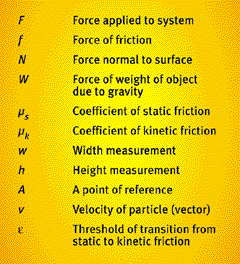Table 1. A summary of notations used in this article.

How is it possible for a smooth book on a smooth table to create a force that resists your efforts to push it? Well, it turns out that even relatively smooth surfaces are actually pretty rough if you look closely enough. It's this roughness that opposes your efforts. But even more interesting is the fact that on a smaller scale, when objects rest against each other, atomic bonds tend to form between them. These bonds form a kind of glue that makes it necessary to apply extra force to get an object moving.

It's possible to measure the effect of this roughness. In fact, this is exactly what Charles Coulomb did in the late eighteenth century. He established a theory of dry friction (since called Coulomb friction) that predicts the maximum friction forces that are exerted on an object in contact with a dry surface before that object moves and becomes dynamic. The theory also predicts the friction forces that the surfaces exert when they are in motion relative to each other.

Don't Give Me No Static

When you are applying force on the book, the friction force opposes your efforts. Let's take a look at a diagram of this situation. Figure 1 shows a free body diagram of the book in static equilibrium, meaning that the book is not moving.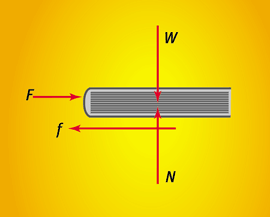Figure 1. A book in a state of static equilibrium.

Since the book is in static equilibrium, we can determine a number of things via the principles of statics. The normal force, N, to the collision of the book with the surface is equal in magnitude to the weight of the book, W. Also, the friction force, f, must also be equal in magnitude to the force being applied on the book, F.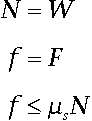(Coulomb Static Friction)

The Coulomb static friction model states that the magnitude of the friction force is less than or equal to the normal force, N, multiplied by a constant coefficient of static friction, ms. This coefficient describes the degree of smoothness between the two surfaces and generally depends on the material composition of the contacting objects. This value typically varies from 0 (which would be a perfectly smooth, frictionless surface) to 1 (for a very rough surface). Some examples of coefficients of static friction can be seen in Table 2.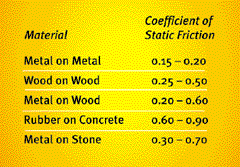Table 2. Some coefficients of static friction.

There are some circumstances where ms can actually be greater than 1. Drag racing tires, for example, are designed to be sticky so that the friction force they exert is greater then the normal force exerted by the road.

When the force you are applying on the book causes the book to be on the verge of sliding, the friction force that opposes your efforts is at its maximum. At this point, slip is said to be impending. Through statics you can calculate the magnitude of the force necessary to cause this slip.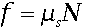(Coulomb static friction model)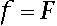(Objects are in static equilibrium)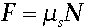(The maximum F before a slip occurs)

Therefore, the maximum force that can be applied on the book before it begins to slip is µsN. What is interesting, and complicated, about static friction is the fact that the friction force increases to equal the applied force until this threshold has been reached.

What Happens Then?

Once the applied force is greater than the slip threshold, the object starts moving. We now leave the world of statics and enter the world of dynamics. It's actually very similar to static friction. The magnitude of the friction force between two dry contacting surfaces that are sliding relative to each other is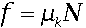where µk is the coefficient of kinetic friction. This force resists the motion of the two bodies. Its direction is opposite the vector of relative velocity between the objects. In general, the value of µk is smaller than ms. However, this does not always have to be the case.

That covers the Coulomb dry friction model in both static and dynamic situations. By simply implementing these two methods, you can create a world represented by interesting physical properties.

How's This Good For Games?

An obvious application of the Coulomb dry friction model is for travel over surfaces. You may have a game that requires a character to travel over various types of terrain. By specifying different coefficients of friction for different types of terrain (asphalt, grass, ice, and so on), you can simulate movement over this terrain in a realistic, and even more importantly, a physically consistent manner.

Many games simulate friction simply by reducing the velocity by a percentage based on the surface type. This may seem at first to be the same thing as the dry friction model described above. However, it differs from it in many critical ways. By adjusting the velocity directly, you eliminate the side effects of applying the friction as a force. These side effects are what make objects in the physical simulation behave the way players expect them to behave. These small breakdowns in the simulation make it glaringly apparent that the world is fake. Perhaps an example would help here.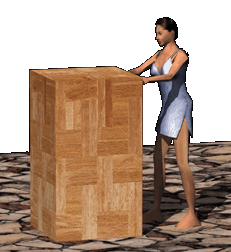Figure 3. You can control how much force Sara must exert on the box before it moves

Say I'm creating an adventure game starring a beautiful woman named Sara running around a dangerous, mystical temple in a stunning cocktail dress. To escape from the temple, Sara must manipulate a series of wooden boxes to activate various switches embedded in the floor. (Don't blame me, my producer came up with the concept.)

Sara pushes the boxes around by applying a horizontal force to the objects. If I do not consider friction at all, then once the boxes are sliding they will slide all around the room, bouncing off the walls forever. Clearly something needs to be done. So, I simply reduce the velocity of the object as it slides around. This can be made to look pretty good. However, there is still a problem.

If you have ever pushed a box really hard, particularly if your point of contact is near the top of the box, the box will sometimes tip over before it starts sliding. In fact, if you throw a box across the room, once it hits the floor it will tumble all over the place instead of simply sliding to a halt. People are used to these facts. They live with them every day. If your world does not address these behaviors, it will not feel right.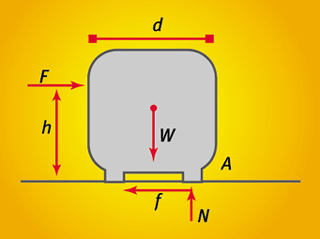Figure 2. Forces exerted on a box as it verges on tipping over.

But why does the box tip over? Well, guess what, it is all about friction. Take a look at the box in Figure 2. Sara will be applying a force, F, to the box h units above the ground. What I'm looking for is a state for the system where the box is about to tip over at point A. I can apply the principles of statics to solve this problem. (If you are not familiar with statics, check out the For Futher Info section at the end of this column.) For an object to be in static equilibrium, the sum of all forces and the sum of all moments in the body must equal zero.

When the box is about to tip over, there is only a reaction to the ground at point A. The support on the other side has no reaction to the ground. Therefore, we can state the equilibrium equations. Let me start with the sum of forces.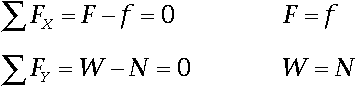The sum of horizontal forces consists only of F and f, and they directly oppose each other. In the vertical direction, the weight W and normal force N are also equal and opposite. The sum of moments however, is a bit more complicated. You may remember from physics that the moment of a force about a point P is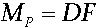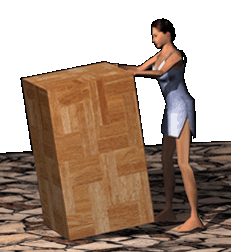Figure 4. Sara tips the box over instead of sliding it away.

where D is the perpendicular distance from the point P to the line of action of the force F. Forces are sliding vectors, meaning that they act equally along their entire line of action. Let's look back at the drawing in Figure 2. When the object is about to tip over, it makes sense to look at the sum of moments about the point A. There are two moments being applied about point A. The force Sara is applying, F, and the force of the weight of the object, W.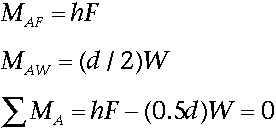At the point of equilibrium where the box is about to slip,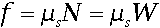So, I can substitute leaving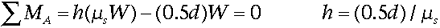If Sara applies the force at a point (0.5d)/ µs units high or higher on the box, the box is going to tip over before it starts sliding. What's even more interesting is the fact that the equation above states that the value for h is not dependent on anything other than the dimensions of the box and the coefficient of static friction. The magnitude of the force F does not matter at all. It may seem that if Sara pushes harder, the box would be more likely to tip. Statics proves that this is not the case.

How Do I Use This Knowledge?

I am convinced. I want to have boxes that tip over if you push them too high. That seems like something cool to have in my game. But how do I go about accomplishing this task?

I have been building up the pieces I need. If you look back to my previous articles, "Collision Response: Bouncy, Trouncy, Fun," and "Lone Game Developer Battles Physics Simulator", I have a soft body dynamics package that models the forces and handles collision with surfaces. I will first handle the kinetic friction problem.

As I described above, the magnitude of the kinetic friction force isand the direction of the force is determined by looking at the current particle velocity. In my simulation, if the velocity of a point is greater than a certain threshold, e, I determine that I need to use static friction for all contacting points. Listing 1 shows the code for calculating and adding in the force of friction.

Listing 1. Code for calculating and adding in friction.

// Calculate Magnitude of Fn
FdotN = DotProduct(&curParticle->contactN,&curParticle->f);

// Calculate Vt Velocity Tangent to Contact Normal
VdotN = DotProduct(&curParticle->contactN,&curParticle->v);
ScaleVector(&curParticle->contactN, VdotN, &Vn);
VectorDifference(&curParticle->v, &Vn, &Vt);

NormalizeVector(&Vt); // Get the Direction of Vt
// Multiply By Normal force magnitude and Coef of Kinetic Friction
ScaleVector(&Vt, (FdotN * m_Ckf), &Vt);

// Add into the Force Accumulator
VectorSum(&curParticle->f,&Vt,&curParticle->f);

The only change I really had to make to the structure of the program was to a storage space for the contact normal for contacting particles.

Static Friction

Handling static friction, however, is much more complicated. The problem is that static friction requires that I determine when each contacting particle makes the transition to sliding. From the calculations above, I know that the point of transition is when

F = µsN. Until that transition occurs, the static friction force needs to prevent sliding completely. That is, I need to make sure that the particle acceleration is kept at zero. Once the particle begins sliding, then the force opposes the acceleration and has a maximum of µsN. All of these conditions lead to a situation that is too complex to be calculated in my simulation. David Baraff (see For Further Info) suggests a couple of approximations.

The more complicated method Baraff suggests is to approach static friction as a quadratic programming problem. However, this method is prone to failure in certain circumstances. The other suggestion, fortunately, is easy to implement.

First, establish a velocity threshold value e which determines when to use static friction. This threshold is then used to scale the friction force as the velocity varies from 0 to this threshold. The formula for calculating the static friction force then becomes

F = (µsN)(v/e). This force is applied in the direction opposite the velocity of the particle. Listing 2 contains the code for handling the static friction forces.

Listing 2. Code for handling static friction forces.

// Calculating Magnitude of Fn
FdotN = DotProduct(&curParticle->contactN,&curParticle->f);

// Calculating Vt Velocity Tangent to Contact Normal
VdotN = DotProduct(&curParticle->contactN,&curParticle->v);
ScaleVector(&curParticle->contactN, VdotN, &Vn);
VectorDifference(&curParticle->v, &Vn, &Vt);
Vmag = VectorSquaredLength(&Vt);
NormalizeVector(&Vt); // Get the Direction of Vt
if (Vmag > STATIC_THRESHOLD) // Handle Static Friction
{
ScaleVector(&Vt, (FdotN * m_Ckf), &Vt);
// Multiply By Normal force magnitude and Coef of Kinetic Friction
VectorSum(&curParticle->f,&Vt,&curParticle->f);
}
else // Handle it as Kinetic Friction
{
Vmag = Vmag / STATIC_THRESHOLD;
// Multiply By Normal force magnitude and Coef of Static // Friction
// And Static approximation ratio
ScaleVector(&Vt, (FdotN * m_Csf * Vmag), &Vt);
VectorSum(&curParticle->f,&Vt,&curParticle->f);
}

In order for this static friction approximation to work, the particle must build up some velocity in order for the static force to kick in. If the value of e is too large, it can cause the object to crawl around a little. By reducing this value, the crawling effect can be eliminated.

One unfortunate side effect of this approximation of static friction is that it can play hell with your integrator. When the particle is moving and subject to kinetic friction, things work well. However, when static friction kicks in, the direction of the static friction force swings wildly with small fluctuations in velocity. This plays havoc with the integration. If the value for e is too small, the differential equations can become "stiff," requiring more complex integration techniques (See "Lone Game Developer Battles Physics Simulator").

Let's Drag

Now I can get objects to tumble around realistically as well as slow to a halt based on the current coefficients of friction. You can download the source code and executable to the sample application from the Game Developer web site (http://www.gdmag.com).

When not fighting the friction that keeps his butt planted in Redondo Beach, Jeff creates custom 3D real-time graphics applications at Darwin 3D. What's the roughest surface you know? E-mail it to him at [email protected].

For Further Info

Baraff, David. "Coping with Friction for Non-Penetrating Rigid Body Simulation," Siggraph Proceedings: July 1991, pp. 31-40.

Beer and Johnston. Vector Mechanics for Engineers: Statics, Sixth Ed. New York: WCB/McGraw-Hill, 1997.

Hecker, Chris. "Behind the Screen" columns. Game Developer, October 1996-June 1997. Also available on Chris's web site at http://www.d6.com.

Lötstedt, P. "Numerical Simulation of Time-Dependent Contact Friction Problems in Rigid Body Mechanics." SIAM Journal of Scientific Statistical Computing Vol. 5, No. 2 (June 1984):
pp. 370-393.

### Latest Jobs

#### Infinity Ward

Woodland Hills, California
11.3.21
Sr. Multiplayer Design Scripter/Programmer

#### Disbelief

Cambridge, Massachusetts
11.3.21
Jr. Programmer

#### 4A Games

Malta
11.3.21
Lead Game Systems Designer New IP

#### XSEED

Torrance, California
11.3.21
More Jobs